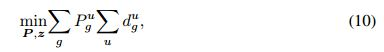# Multiple Variables in Objective Function

I have this optimization probemto solve. Its a mix integer programming problem. However, I don’t know how to use two or more variables in the objective function. I have used this code

sum_of_Pgu_over_g = sum(P_gu,1);
sum_of_departuregu_over_u = sum(departure_gu,2);
cvx_begin
variables P_gu Z sum_of_Pgu_over_g sum_of_departuregu_over_u;
dual variable lambda1 lambda2 lambda3 lambda4 lambda5 lambda6;
minimize P_gu Z sum_of_Pgu_over_g sum_of_departuregu_over_u
subject to
cvx_end`

It gives me the error in the objective function line “Error: Unexpected MATLAB expression.”, due to which I cannot proceed.

Can you please provide complete reproducible code, and use the preformatted text button, so that your code appears exactly as entered, and can be copied and pasted. I don’t really know what code you entered, and therefore am not sure exactly what you did wrong. You can edit the original post if you want.

if you really have a mixed integer programming problem, you will need a binary or integer declaration somewhere. What are you trying to do with dual variables? I suppose you didn’t show the constraints because you already got an error in the objective function?

I have no idea what your problem is or what your code is supposed to represent.

Where does x appear in the depicted objective function? Does d depend on x? You need o spell out much more clearly what the optimization problem is which you would like to represent in CVX.

You can not have a minimize statement consisting of 2 different variables separated by spaces. Do you mean for the two variables to be multiplied together? If so, that is not allowed.

Are you sure you problem is (mixed integer) convex? Why isn’t CVX accepting my model? READ THIS FIRST!

Ok, so the P in the problem is the power required for the device to transmit data. d_gu is the departure time of packet from cluster to the base station. Z is the number of resource blocks assigned to each base station. P & P_gu are the same matrices.
Values of these matrices/vectors are:

``````P = P_gu = [0.1250 0.1250 0.1250; 0.1250 0.1250 0.1250; 0.1250 0.1250 0.1250]
Z = [ 1     5     2     6    10]
d_gu = [1.4e-06 1.5e-13 3.5e-05; 1.4e-06 1.5e-13 3.5e-05; 1.4e-06 1.5e-13 3.5e-05]
Power = P_gu;
resourceblocks = Z;
sum_of_Pgu_over_g = sum(P_gu,1);
sum_of_departuregu_over_u = sum(departure_gu,2);
cvx_begin
variables Power resourceblocks sum_of_Pgu_over_g sum_of_departuregu_over_u;
dual variable lambda1;
minimize norm(Power) + norm(resourceblocks) + norm(sum_of_Pgu_over_g) + norm(sum_of_departuregu_over_u)
subject to
lambda1: sum(Z,1) <= 25;
cvx_end
``````

The objective function is a mixed integer programming problem. I just want to know if I can take the norms of these matrices/vectors in order to find their optimum value. And if it is the correct approach, how will I know the optimum value of resources to be used or power to be allocated?

Thank you very much for your time and consideration.

When you declare a CVX variable, that variable has no relation to a previously defined MATLAB variable of the same name. Your objective function either consists of norm of CVX variable, not tied or constrained to anything else, or is the norm of a constant (i.e., MATLAB variable, not CVX variable), and therefore not affecting the argmin. Your supposed constraint on Z is not a CVX constraint, because it only involves a MATLAB variablle, and not a CVX variable or expression; and so it will evaluate to vector of 0 and 1 (in this case all 1 given your Z), but have no bearing on your optimization problem.

You are allowed to use norm as you have, but U doubt it is doing what you want or need.

You may need modeling help, which you should obtain elsewhere. And I think you need to carefully read the CVX Users’ Guide. I think you need to better learn the basics of MATLAB as well, which you should probably do before trying to learn CVX.